# Back to Collections Lists Array List Linked List

• Slides: 29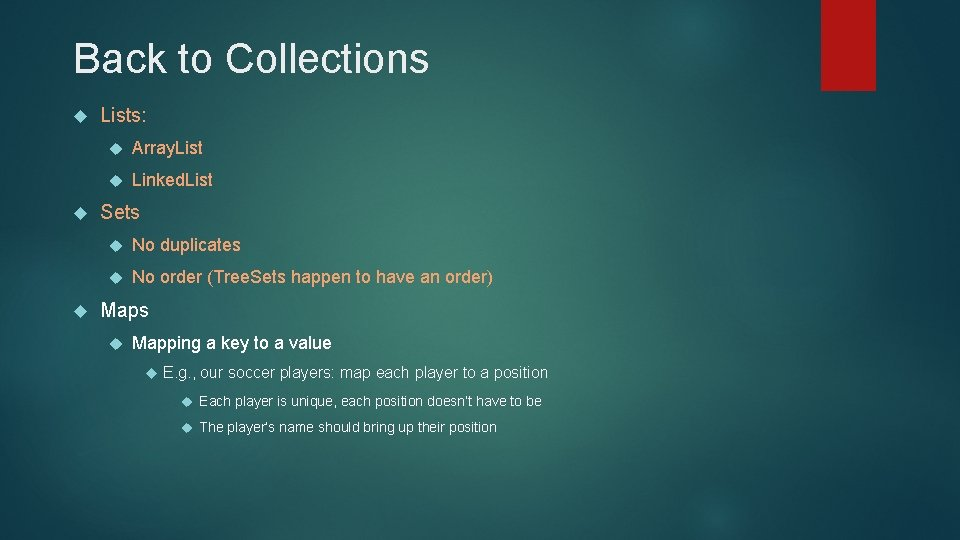Back to Collections Lists: Array. List Linked. List Sets No duplicates No order (Tree. Sets happen to have an order) Maps Mapping a key to a value E. g. , our soccer players: map each player to a position Each player is unique, each position doesn’t have to be The player’s name should bring up their position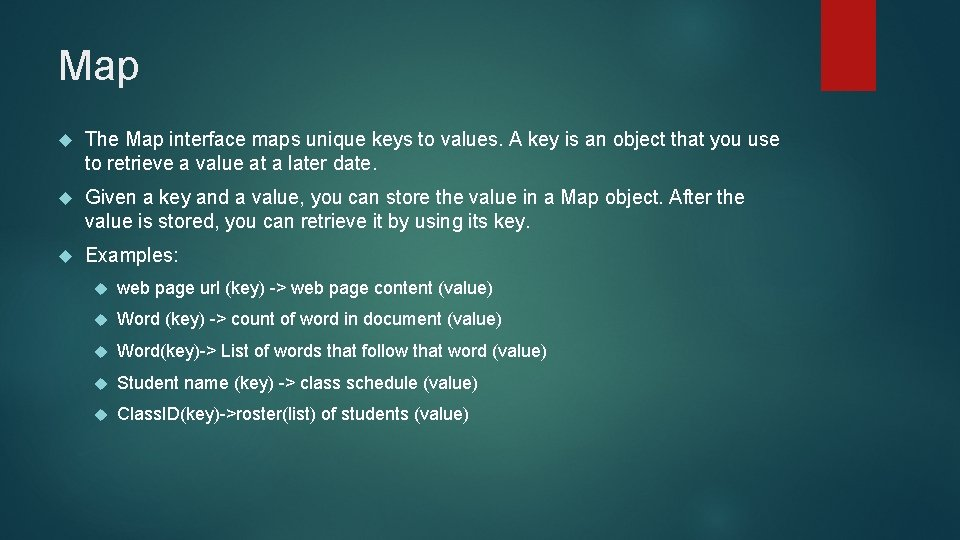Map The Map interface maps unique keys to values. A key is an object that you use to retrieve a value at a later date. Given a key and a value, you can store the value in a Map object. After the value is stored, you can retrieve it by using its key. Examples: web page url (key) -> web page content (value) Word (key) -> count of word in document (value) Word(key)-> List of words that follow that word (value) Student name (key) -> class schedule (value) Class. ID(key)->roster(list) of students (value)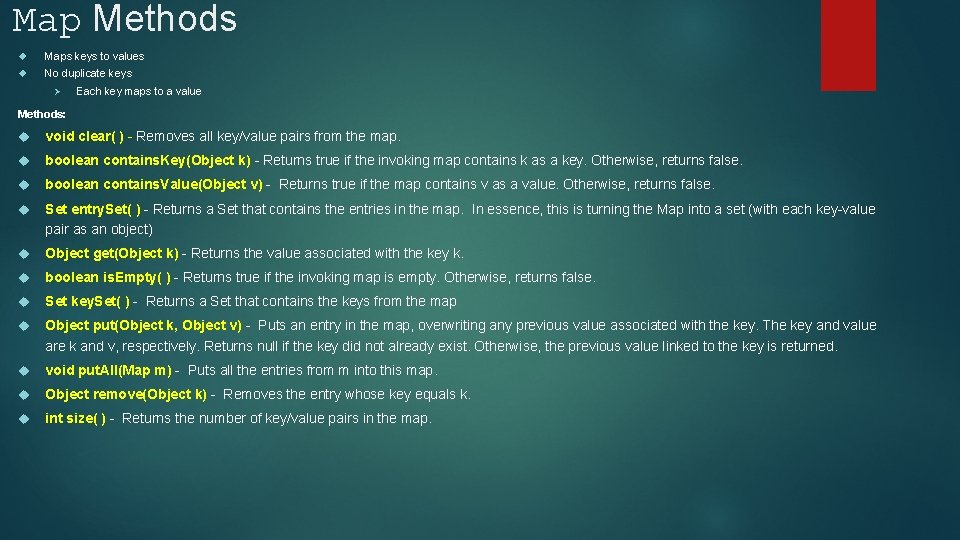Map Methods Maps keys to values No duplicate keys Ø Each key maps to a value Methods: void clear( ) - Removes all key/value pairs from the map. boolean contains. Key(Object k) - Returns true if the invoking map contains k as a key. Otherwise, returns false. boolean contains. Value(Object v) - Returns true if the map contains v as a value. Otherwise, returns false. Set entry. Set( ) - Returns a Set that contains the entries in the map. In essence, this is turning the Map into a set (with each key-value pair as an object) Object get(Object k) - Returns the value associated with the key k. boolean is. Empty( ) - Returns true if the invoking map is empty. Otherwise, returns false. Set key. Set( ) - Returns a Set that contains the keys from the map Object put(Object k, Object v) - Puts an entry in the map, overwriting any previous value associated with the key. The key and value are k and v, respectively. Returns null if the key did not already exist. Otherwise, the previous value linked to the key is returned. void put. All(Map m) - Puts all the entries from m into this map. Object remove(Object k) - Removes the entry whose key equals k. int size( ) - Returns the number of key/value pairs in the map.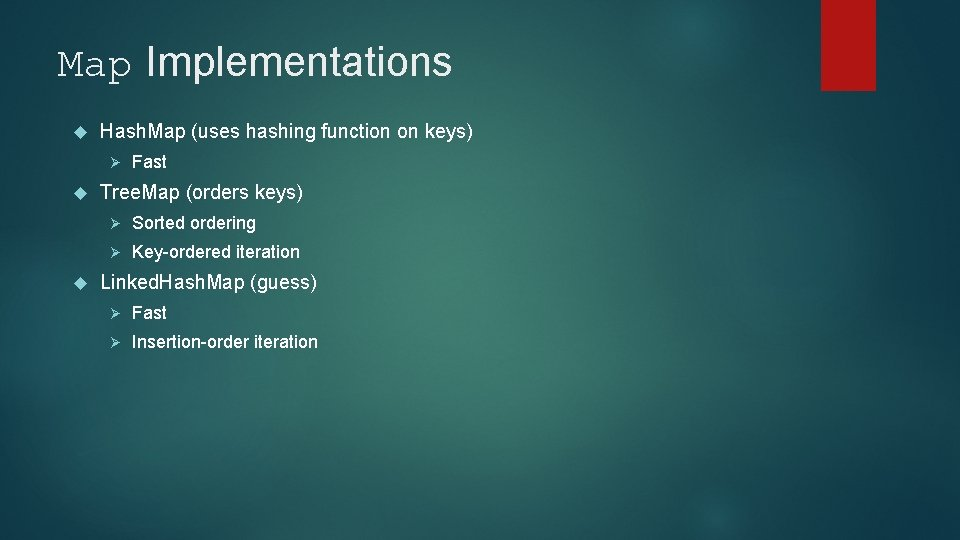Map Implementations Hash. Map (uses hashing function on keys) Ø Fast Tree. Map (orders keys) Ø Sorted ordering Ø Key-ordered iteration Linked. Hash. Map (guess) Ø Fast Ø Insertion-order iteration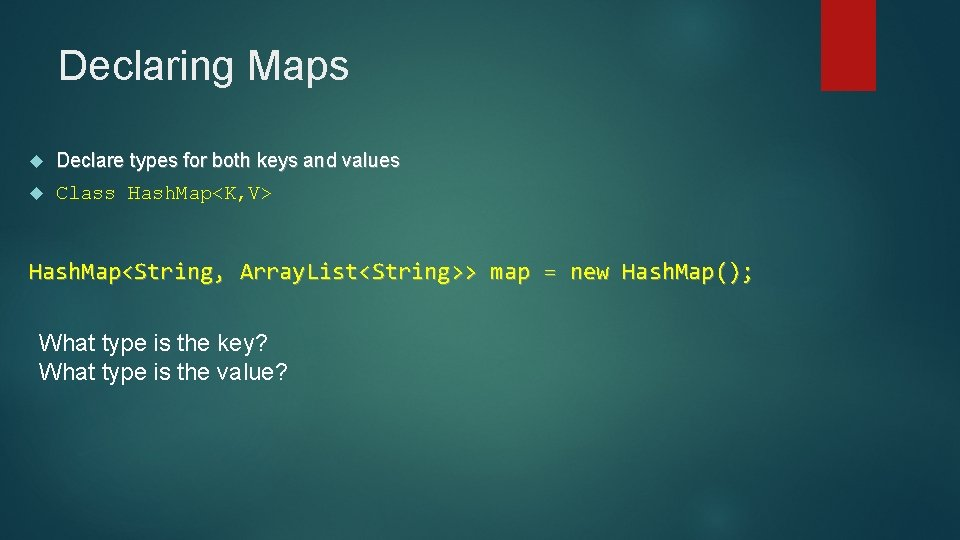Declaring Maps Declare types for both keys and values Class Hash. Map<K, V> Hash. Map<String, Array. List<String>> map = new Hash. Map(); What type is the key? What type is the value?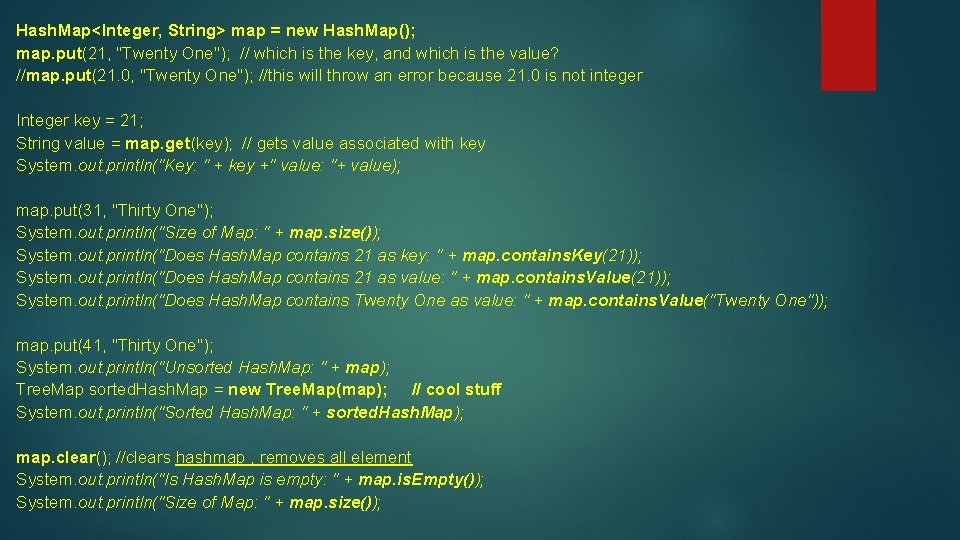Hash. Map<Integer, String> map = new Hash. Map(); map. put(21, "Twenty One"); // which is the key, and which is the value? //map. put(21. 0, "Twenty One"); //this will throw an error because 21. 0 is not integer Integer key = 21; String value = map. get(key); // gets value associated with key System. out. println("Key: " + key +" value: "+ value); map. put(31, "Thirty One"); System. out. println("Size of Map: " + map. size()); System. out. println("Does Hash. Map contains 21 as key: " + map. contains. Key(21)); System. out. println("Does Hash. Map contains 21 as value: " + map. contains. Value(21)); System. out. println("Does Hash. Map contains Twenty One as value: " + map. contains. Value("Twenty One")); map. put(41, "Thirty One"); System. out. println("Unsorted Hash. Map: " + map); Tree. Map sorted. Hash. Map = new Tree. Map(map); // cool stuff System. out. println("Sorted Hash. Map: " + sorted. Hash. Map); map. clear(); //clears hashmap , removes all element System. out. println("Is Hash. Map is empty: " + map. is. Empty()); System. out. println("Size of Map: " + map. size());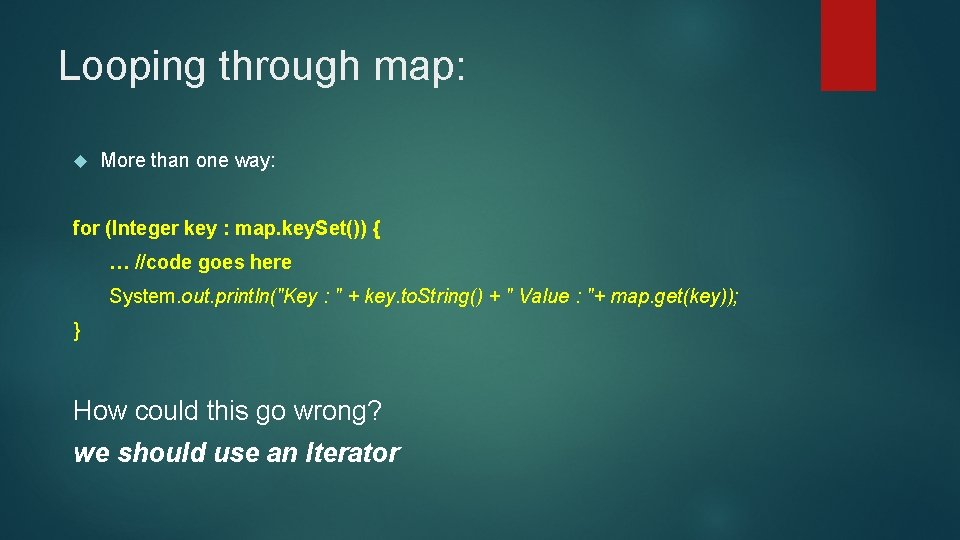Looping through map: More than one way: for (Integer key : map. key. Set()) { … //code goes here System. out. println("Key : " + key. to. String() + " Value : "+ map. get(key)); } How could this go wrong? we should use an Iterator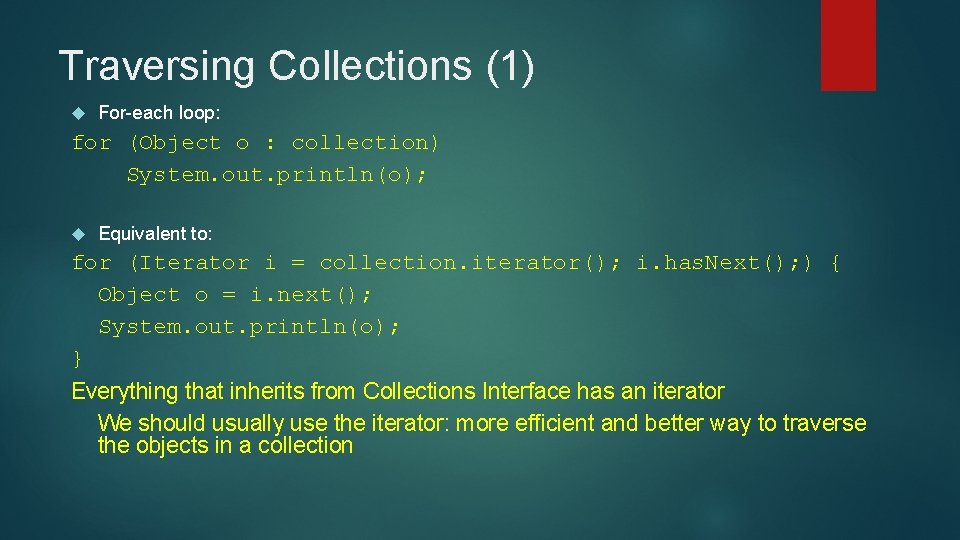Traversing Collections (1) For-each loop: for (Object o : collection) System. out. println(o); Equivalent to: for (Iterator i = collection. iterator(); i. has. Next(); ) { Object o = i. next(); System. out. println(o); } Everything that inherits from Collections Interface has an iterator We should usually use the iterator: more efficient and better way to traverse the objects in a collectionHow to use an iterator: Iterator enables you to cycle through a collection List. Iterator extends Iterator to allow bidirectional traversal of a list obtaining or removing elements Can go backwards and forward in list. To use an iterator: Obtain an iterator (pointer) to the start of the collection Set up a loop that makes a call to has. Next( ) call the collection's iterator( ) method. Have the loop iterate as long as has. Next( ) returns true. Within the loop, obtain each element by calling next( ).Traversing Collections: Iterators Java Interface Object next() boolean has. Next() get the next element are there more elements? void remove() removes the previous element Only safe way to remove elements during iteration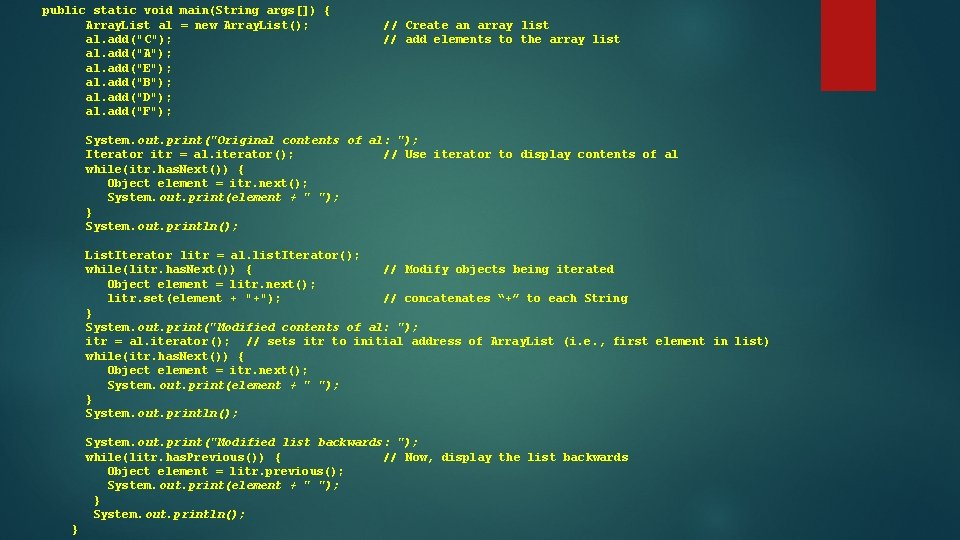public static void main(String args[]) { Array. List al = new Array. List(); al. add("C"); al. add("A"); al. add("E"); al. add("B"); al. add("D"); al. add("F"); // Create an array list // add elements to the array list System. out. print("Original contents of al: "); Iterator itr = al. iterator(); // Use iterator to display contents of al while(itr. has. Next()) { Object element = itr. next(); System. out. print(element + " "); } System. out. println(); List. Iterator litr = al. list. Iterator(); while(litr. has. Next()) { // Modify objects being iterated Object element = litr. next(); litr. set(element + "+"); // concatenates “+” to each String } System. out. print("Modified contents of al: "); itr = al. iterator(); // sets itr to initial address of Array. List (i. e. , first element in list) while(itr. has. Next()) { Object element = itr. next(); System. out. print(element + " "); } System. out. println(); System. out. print("Modified list backwards: "); while(litr. has. Previous()) { // Now, display the list backwards Object element = litr. previous(); System. out. print(element + " "); } System. out. println(); }List. Iterator methods: void add(Object obj) Inserts obj into the list in front of the element that will be returned by the next call to next( ). boolean has. Next( ) Returns true if there is a next element. Otherwise, returns false. boolean has. Previous( ) Returns true if there is a previous element. Otherwise, returns false. Object next( ) Returns the next element. A No. Such. Element. Exception is thrown if there is not a next element. int next. Index( ) Returns the index of the next element. If there is not a next element, returns the size of the list. Object previous( ) Returns the previous element. A No. Such. Element. Exception is thrown if there is not a previous element. int previous. Index( ) Returns the index of the previous element. If there is not a previous element, returns -1. void remove( ) Removes the current element from the list. An Illegal. State. Exception is thrown if remove( ) is called before next( ) or previous( ) is invoked. void set(Object obj) Assigns obj to the current element. This is the element last returned by a call to either next( ) or previous( ).Filter Algorithm void filter(Collection c) { Iterator i = c. iterator(); while( i. has. Next() ) { // if the next element does not // adhere to the condition, remove it if (!cond(i. next())) { i. remove(); } } }Tree. Sets: How do Tree. Sets work? What makes Tree. Sets unique compared to Hash. Sets? What if we put student objects into the tree? How about car objects?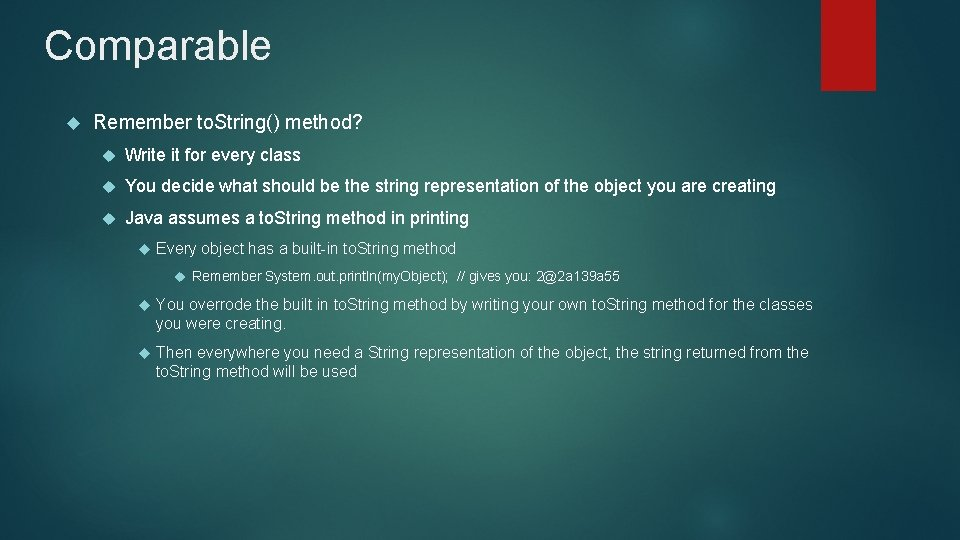Comparable Remember to. String() method? Write it for every class You decide what should be the string representation of the object you are creating Java assumes a to. String method in printing Every object has a built-in to. String method Remember System. out. println(my. Object); // gives you: [email protected] a 139 a 55 You overrode the built in to. String method by writing your own to. String method for the classes you were creating. Then everywhere you need a String representation of the object, the string returned from the to. String method will be usedComparables You now get to do something similar for comparing objects: You decide what will make one object greater than, less than, or equal to another object of the same class. So you decide if you want to compare students by last name, by lab score, by total score, or whatever you want.public class Student 2 implements Comparable<Student 2> { String f, l; int g 1, g 2, g 3; int total; public Student 2(String f 1, String l 1, int g 11, int g 21, int g 31) { f = f 1; l = l 1; g 1 = g 11; g 2 = g 21; g 3 = g 31; total = get. Total(); } public int compare. To(Student 2 s 2) { System. out. println(total + " " + s 2. get. Total()); if (total > s 2. get. Total()) { return 1; } else if (total < s 2. get. Total()) { return -1; } else { return 0; } } } private int get. Total() { return(g 1 + g 2 + g 3); public String to. String() { String s = ""; s += l + " "+f+": "+total; return(s); } }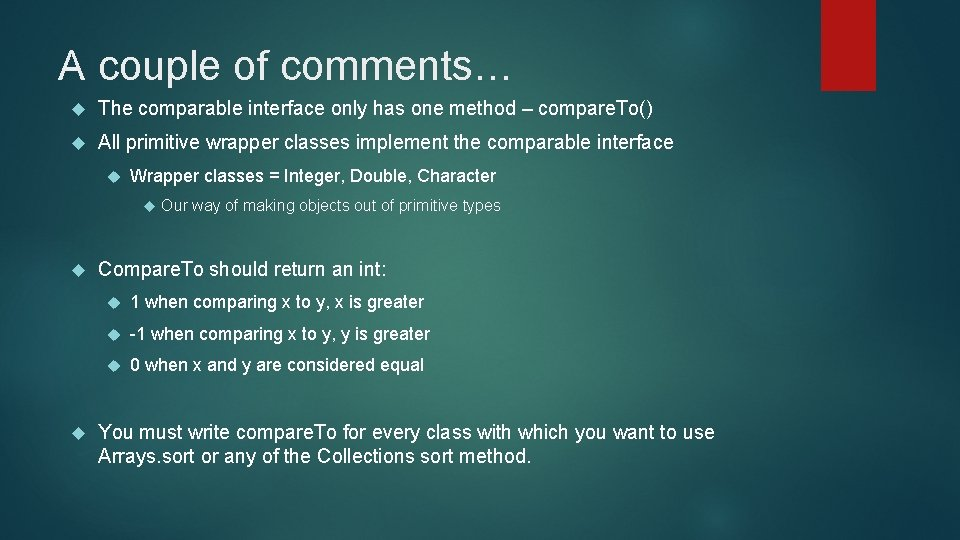A couple of comments… The comparable interface only has one method – compare. To() All primitive wrapper classes implement the comparable interface Wrapper classes = Integer, Double, Character Our way of making objects out of primitive types Compare. To should return an int: 1 when comparing x to y, x is greater -1 when comparing x to y, y is greater 0 when x and y are considered equal You must write compare. To for every class with which you want to use Arrays. sort or any of the Collections sort method.public static void main(String[] args) { Student 2 s = new Student 2("Harry", "Jones", 20, 20); Student 2 s 2 = new Student 2("Anne", "Rivers", 50, 50); if (s. compare. To(s 2)==1) { System. out. println("s is greater "); } else if (s. compare. To(s 2)==-1) { System. out. println("s 2 is greater"); } else { System. out. println("equal"); } 60 150 s 2 is greater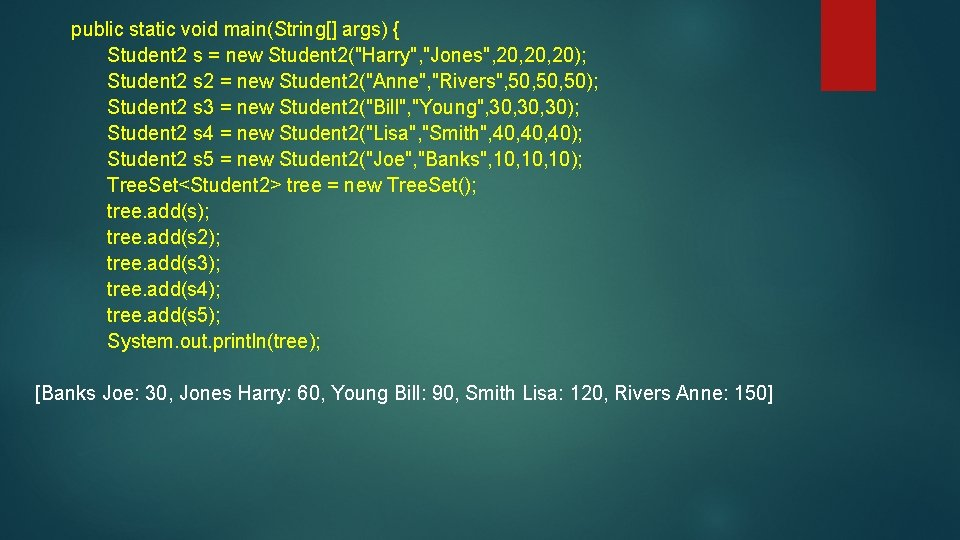public static void main(String[] args) { Student 2 s = new Student 2("Harry", "Jones", 20, 20); Student 2 s 2 = new Student 2("Anne", "Rivers", 50, 50); Student 2 s 3 = new Student 2("Bill", "Young", 30, 30); Student 2 s 4 = new Student 2("Lisa", "Smith", 40, 40); Student 2 s 5 = new Student 2("Joe", "Banks", 10, 10); Tree. Set<Student 2> tree = new Tree. Set(); tree. add(s 2); tree. add(s 3); tree. add(s 4); tree. add(s 5); System. out. println(tree); [Banks Joe: 30, Jones Harry: 60, Young Bill: 90, Smith Lisa: 120, Rivers Anne: 150]public class Student 2 implements Comparable<Student 2> { String f, l; int g 1, g 2, g 3; int total; public Student 2(String f 1, String l 1, int g 11, int g 21, int g 31) { f = f 1; l = l 1; g 1 = g 11; g 2 = g 21; g 3 = g 31; total = get. Total(); } public int compare. To(Student 2 s 2) { return(l. compare. To(s 2. l)); } } private int get. Total() { return(g 1 + g 2 + g 3); public String to. String() { String s = ""; s += l + " "+f+": "+total; return(s); } }public static void main(String[] args) { Student 2 s = new Student 2("Harry", "Jones", 20, 20); Student 2 s 2 = new Student 2("Anne", "Rivers", 50, 50); Student 2 s 3 = new Student 2("Bill", "Young", 30, 30); Student 2 s 4 = new Student 2("Lisa", "Smith", 40, 40); Student 2 s 5 = new Student 2("Joe", "Banks", 10, 10); Tree. Set<Student 2> tree = new Tree. Set(); tree. add(s 2); tree. add(s 3); tree. add(s 4); tree. add(s 5); System. out. println(tree); [Banks Joe: 30, Jones Harry: 60, Rivers Anne: 150, Smith Lisa: 120, Young Bill: 90]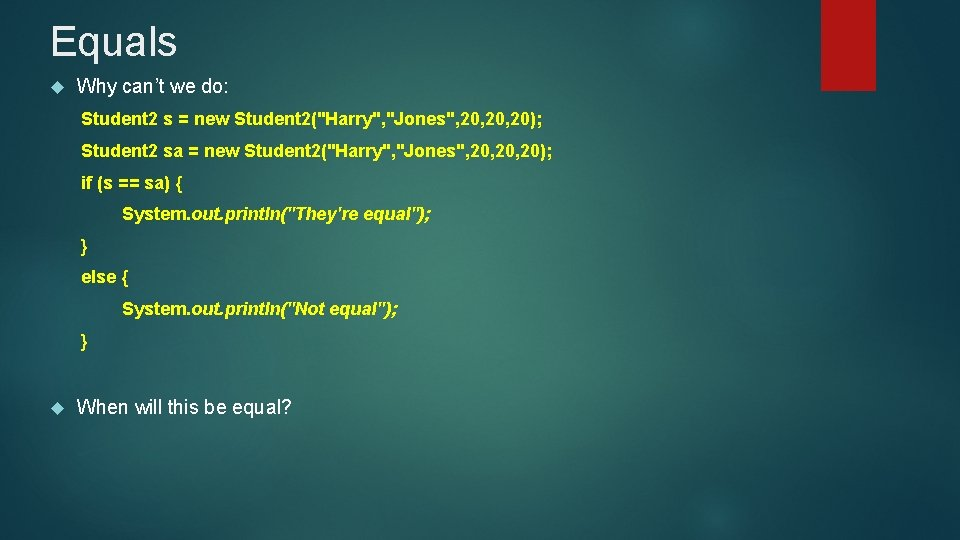Equals Why can’t we do: Student 2 s = new Student 2("Harry", "Jones", 20, 20); Student 2 sa = new Student 2("Harry", "Jones", 20, 20); if (s == sa) { System. out. println("They're equal"); } else { System. out. println("Not equal"); } When will this be equal?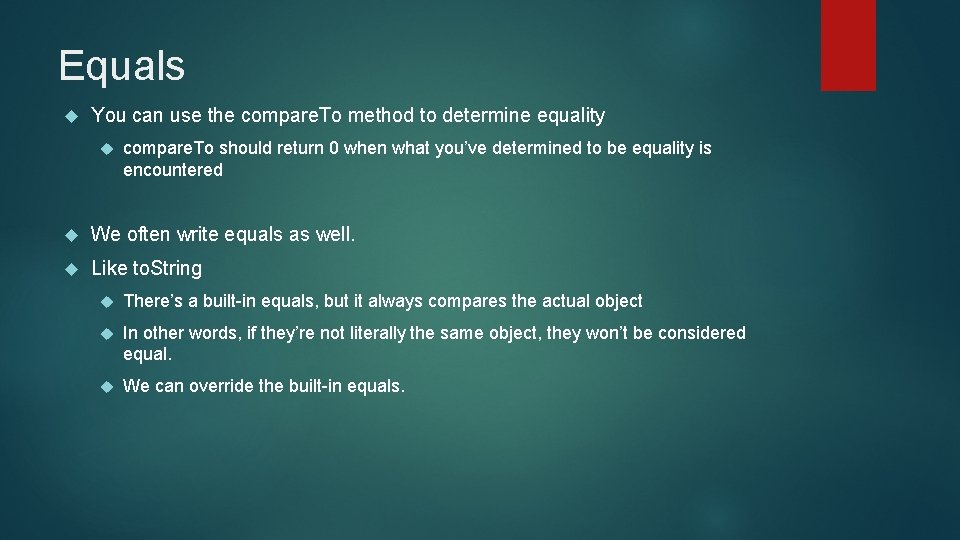Equals You can use the compare. To method to determine equality compare. To should return 0 when what you’ve determined to be equality is encountered We often write equals as well. Like to. String There’s a built-in equals, but it always compares the actual object In other words, if they’re not literally the same object, they won’t be considered equal. We can override the built-in equals.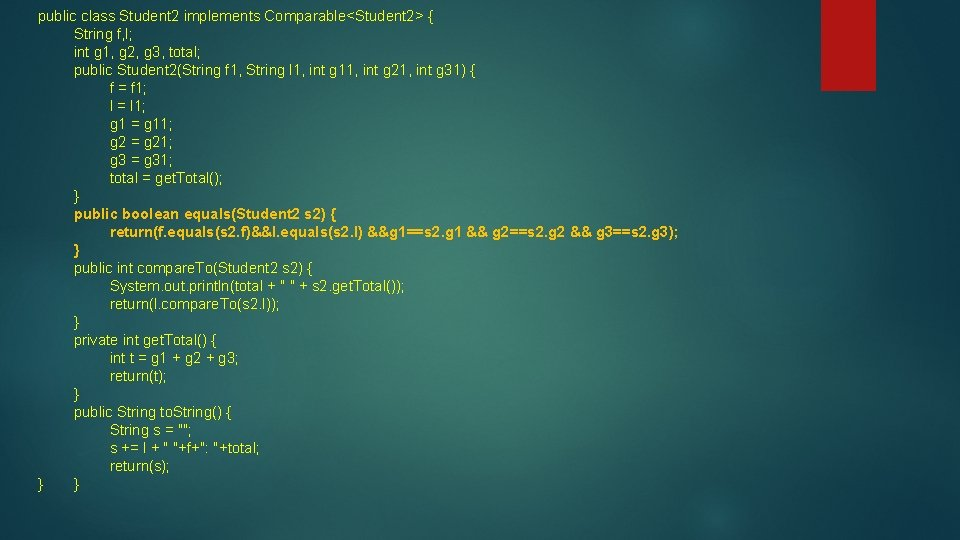public class Student 2 implements Comparable<Student 2> { String f, l; int g 1, g 2, g 3, total; public Student 2(String f 1, String l 1, int g 11, int g 21, int g 31) { f = f 1; l = l 1; g 1 = g 11; g 2 = g 21; g 3 = g 31; total = get. Total(); } public boolean equals(Student 2 s 2) { return(f. equals(s 2. f)&&l. equals(s 2. l) &&g 1==s 2. g 1 && g 2==s 2. g 2 && g 3==s 2. g 3); } public int compare. To(Student 2 s 2) { System. out. println(total + " " + s 2. get. Total()); return(l. compare. To(s 2. l)); } private int get. Total() { int t = g 1 + g 2 + g 3; return(t); } public String to. String() { String s = ""; s += l + " "+f+": "+total; return(s); } }public static void main(String[] args) { Student 2 s = new Student 2("Harry", "Jones", 20, 20); Student 2 sa = new Student 2("Harry", "Jones", 20, 20); if (s == sa) { System. out. println("They're equal"); } else { System. out. println("Not equal"); } if (s. equals(sa)) { System. out. println("they're equal"); } else { System. out. println("nope"); } Not equal they're equal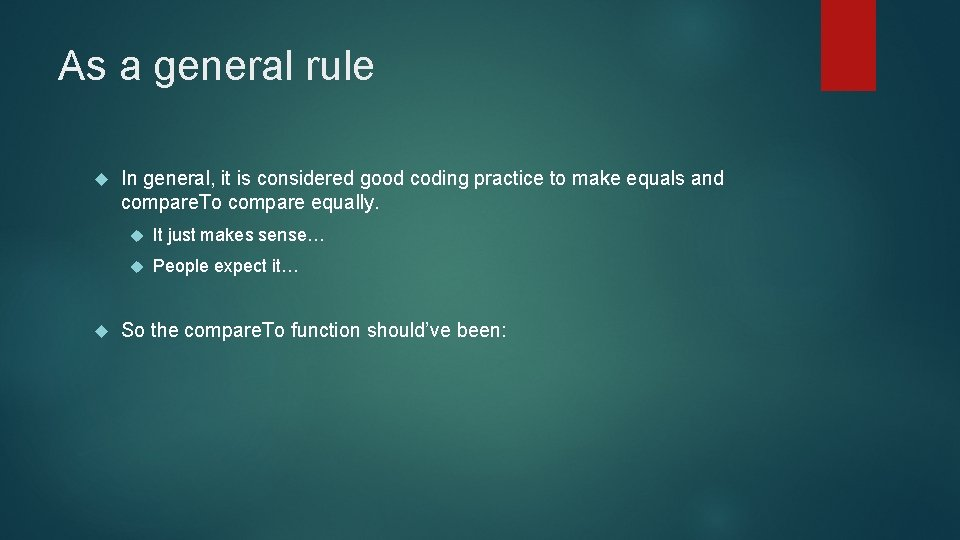As a general rule In general, it is considered good coding practice to make equals and compare. To compare equally. It just makes sense… People expect it… So the compare. To function should’ve been: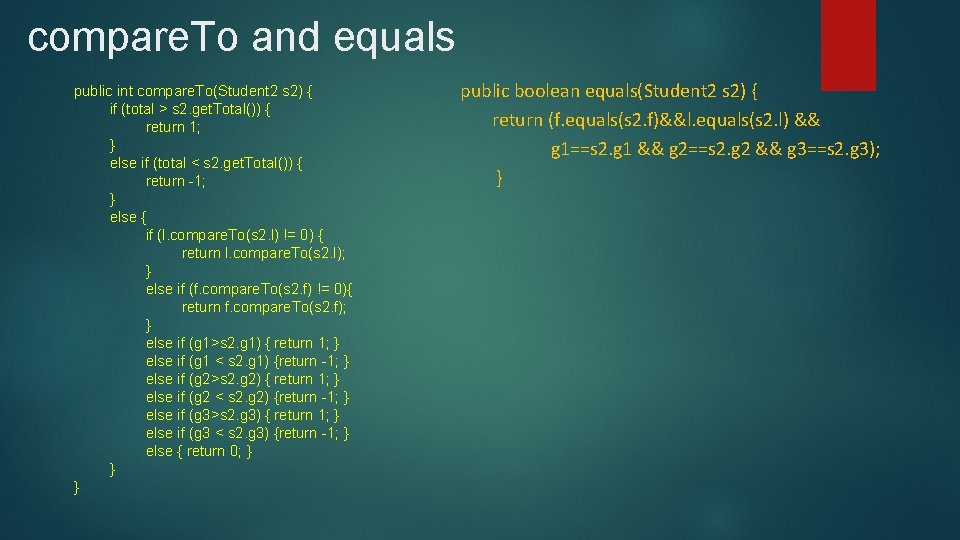compare. To and equals public int compare. To(Student 2 s 2) { if (total > s 2. get. Total()) { return 1; } else if (total < s 2. get. Total()) { return -1; } else { if (l. compare. To(s 2. l) != 0) { return l. compare. To(s 2. l); } else if (f. compare. To(s 2. f) != 0){ return f. compare. To(s 2. f); } else if (g 1>s 2. g 1) { return 1; } else if (g 1 < s 2. g 1) {return -1; } else if (g 2>s 2. g 2) { return 1; } else if (g 2 < s 2. g 2) {return -1; } else if (g 3>s 2. g 3) { return 1; } else if (g 3 < s 2. g 3) {return -1; } else { return 0; } } } public boolean equals(Student 2 s 2) { return (f. equals(s 2. f)&&l. equals(s 2. l) && g 1==s 2. g 1 && g 2==s 2. g 2 && g 3==s 2. g 3); }